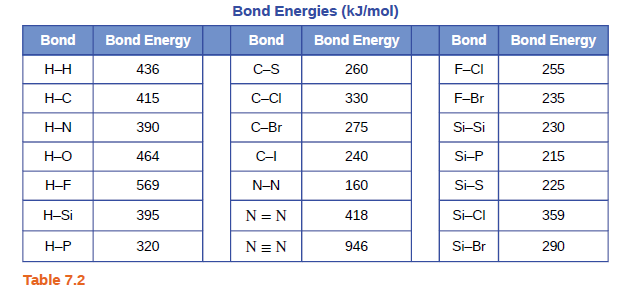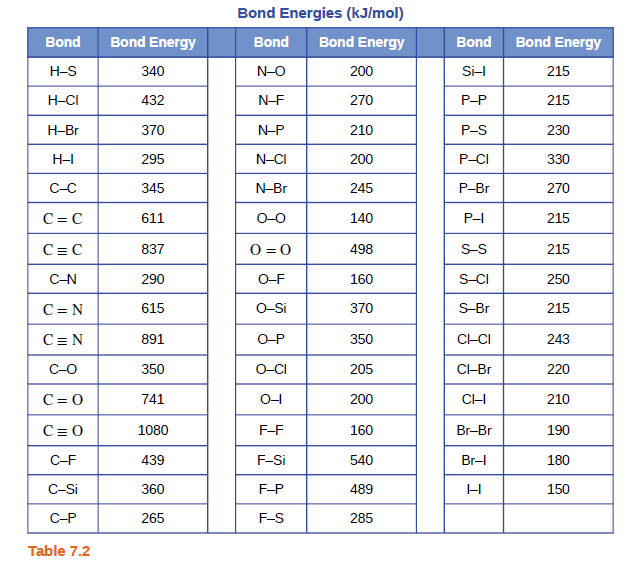# Problem: Using the bond energies in Table 7.2, determine the approximate enthalpy change for each of the following reactions:(c) 2C2H6(g) + 7O2(g) ⟶ 4CO2(g) + 6H2O(g)

🤓 Based on our data, we think this question is relevant for Professor Szatkowski's class at UC.

###### FREE Expert Solution

2C2H6(g) + 7O2(g) ⟶ 4CO2(g) + 6H2O(g)###### Problem Details

Using the bond energies in Table 7.2, determine the approximate enthalpy change for each of the following reactions:
(c) 2C2H6(g) + 7O2(g) ⟶ 4CO2(g) + 6H2O(g)